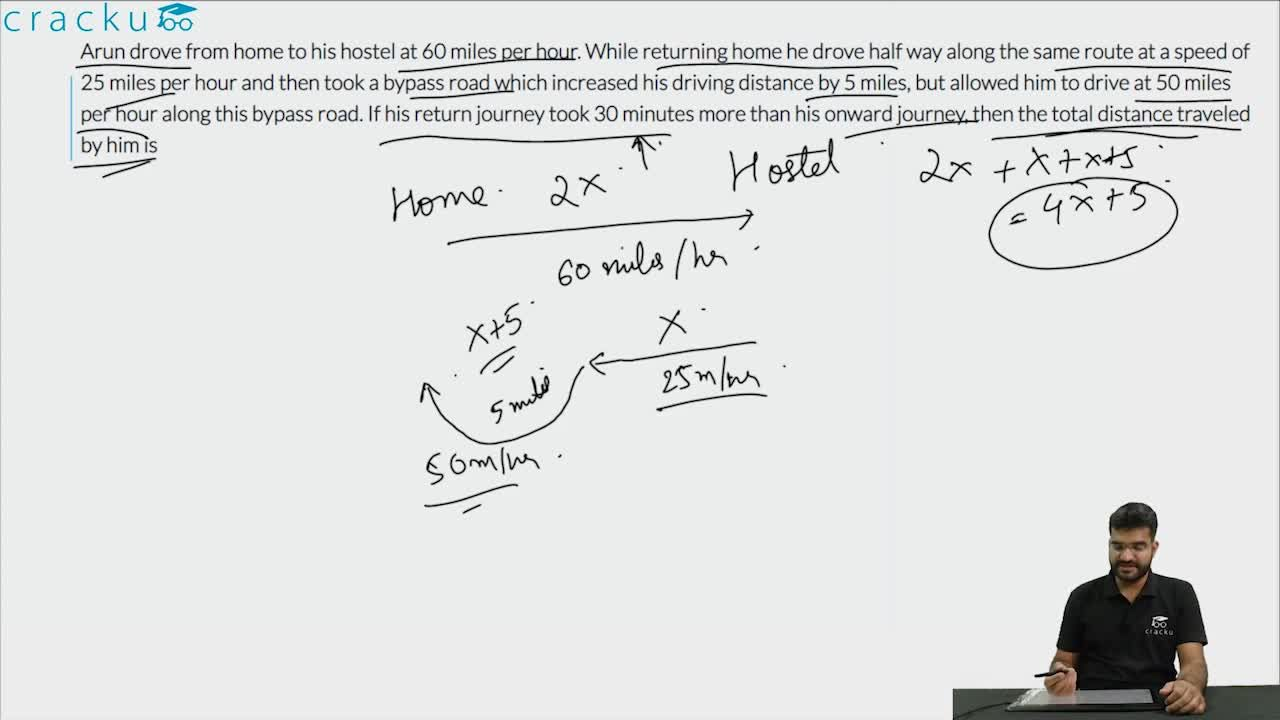Question 70

# Arun drove from home to his hostel at 60 miles per hour. While returning home he drove half way along the same route at a speed of 25 miles per hour and then took a bypass road which increased his driving distance by 5 miles, but allowed him to drive at 50 miles per hour along this bypass road. If his return journey took 30 minutes more than his onward journey, then the total distance traveled by him is

Solution

Let the distance between the home and office be $$2x$$ miles
Time taken for going in the morning = $$\frac{2x}{60}$$ hrs
Time taken for going back in the evening = $$\frac{x}{25} + \frac{x+5}{50}$$. hrs
It is given that he took 30 minutes (0.5 hrs) more in the evening

Hence $$\frac{2x}{60}$$ hrs + 0.5 = $$\frac{x}{25} + \frac{x+5}{50}$$
Solving for x, we get x = 15 miles.
Total distance traveled = 2x + x + x + 5 = 4x + 5 = 65 Miles

### View Video Solution• All Quant CAT Formulas and shortcuts PDF
• 30+ CAT previous papers with solutions PDF

##### Jaswanth Chakka

1 year ago

could you please explain the why this equation having the -x/30?

X/25+X+5/50-X/30 = 1/2

##### Arijit Nandi

3 months, 2 weeks ago

While returning, he is taken more 30 min extra, for that, when equating ( return time - going time) =30 min, so, return time and going time have 30 min gap